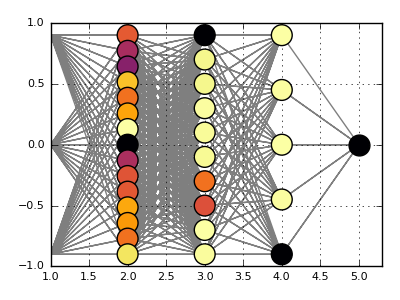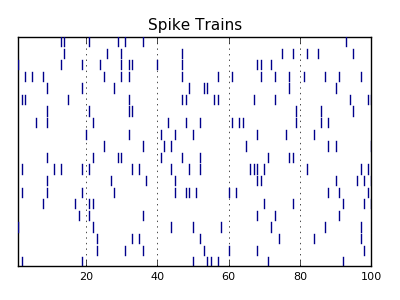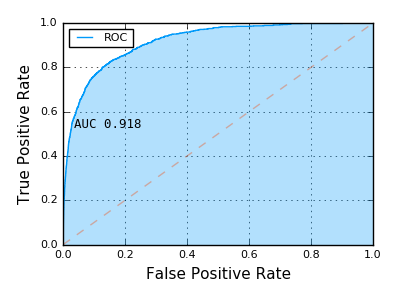dummy-link

23

4

5

3

# MLPlotsCommon plotting recipes for statistics and machine learning.

This package uses RecipesBase to provide mappings from types defined in statistics and machine learning packages to generic descriptions of visualization attributes and data. These recipes can then be used in conjunction with Plots.jl to provide flexible statistical and machine learning visualizations which are independent of both the platform and graphical library.

Many recipes are conditionally included and are loaded on the relevant `using` call of the library. For example `using ROCAnalysis, MLPlots` will load plotting recipes for roc curves defined in ROCAnalysis. Recipes include:

## OnlineAI

Neural nets with OnlineAI.jl. Show the current state of a neural net:

``````using OnlineAI, MLPlots
net = buildClassificationNet(3, 1, [15,10,5])
plot(net)
``````For spiking neuron models, a spike (or raster) plot is useful to see firing times among neurons:

``````n = 20
spikes = SpikeTrains(n, title = "Spike Trains", color = :darkblue)
for t=1:100, i=1:n
if rand() < 0.1
push!(spikes, i, t)
end
end
spikes.plt
``````## ROCAnalysis

ROCAnalysis.jl

``````using ROCAnalysis, MLPlots
curve = ROCAnalysis.roc(2+2randn(1000), -2+2randn(100000))
plot(curve)
``````11/20/2015

22 days ago

69 commits